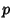## Swinnerton-Dyer Conjecture

In the early 1960s, B. Birch and H. P. F. Swinnerton-Dyer conjectured that if a given Elliptic Curve has an infinite number of solutions, then the associated-function has value 0 at a certain fixed point. In 1976, Coates and Wiles showed that elliptic curves with Complex multiplication having an infinite number of solutions have-functions which are zero at the relevant fixed point (Coates-Wiles Theorem), but they were unable to prove the converse. V. Kolyvagin extended this result to modular curves.

References

Cipra, B. ``Fermat Prover Points to Next Challenges.'' Science 271, 1668-1669, 1996.

Ireland, K. and Rosen, M. ``New Results on the Birch-Swinnerton-Dyer Conjecture.'' §20.5 in A Classical Introduction to Modern Number Theory, 2nd ed. New York: Springer-Verlag, pp. 353-357, 1990.

Mazur, B. and Stevens, G. (Eds.).-Adic Monodromy and the Birch and Swinnerton-Dyer Conjecture. Providence, RI: Amer. Math. Soc., 1994.